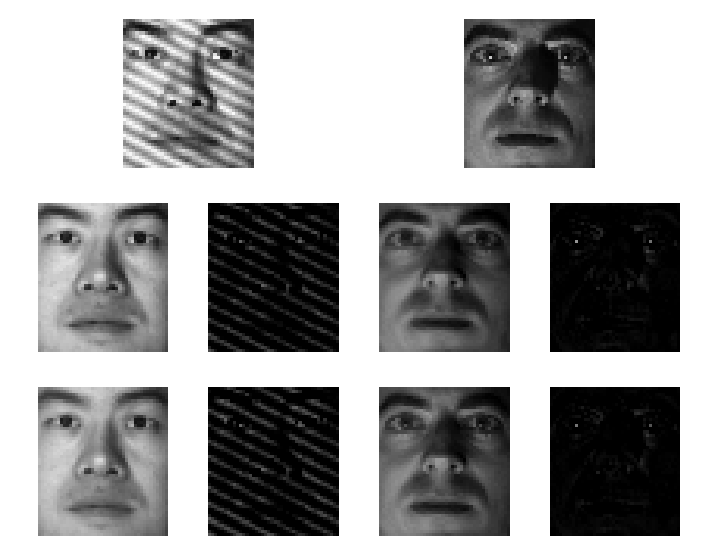# Analysis and Improvement of Low Rank Representation for Subspace segmentation### Abstract

We analyze and improve low rank representation (LRR), the state-of-the-art algorithm for subspace segmentation of data. We prove that for the noiseless case, the optimization model of LRR has a unique solution, which is the shape interaction matrix (SIM) of the data matrix. So in essence LRR is equivalent to factorization methods. We also prove that the minimum value of the optimization model of LRR is equal to the rank of the data matrix. For the noisy case, we show that LRR can be approximated as a factorization method that combines noise removal by column sparse robust PCA. We further propose an improved version of LRR, called Robust Shape Interaction (RSI), which uses the corrected data as the dictionary instead of the noisy data. RSI is more robust than LRR when the corruption in data is heavy. Experiments on both synthetic and real data testify to the improved robustness of RSI.

Type